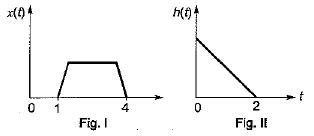Courses

# Test: Introduction to Systems

## 10 Questions MCQ Test Topicwise Question Bank for Electronics Engineering | Test: Introduction to Systems

Description
This mock test of Test: Introduction to Systems for GATE helps you for every GATE entrance exam. This contains 10 Multiple Choice Questions for GATE Test: Introduction to Systems (mcq) to study with solutions a complete question bank. The solved questions answers in this Test: Introduction to Systems quiz give you a good mix of easy questions and tough questions. GATE students definitely take this Test: Introduction to Systems exercise for a better result in the exam. You can find other Test: Introduction to Systems extra questions, long questions & short questions for GATE on EduRev as well by searching above.
QUESTION: 1

Solution:
QUESTION: 2

Solution:
QUESTION: 3

### The unit impulse response of a linear time invariant system is the unit step function u(t) for t > 0, the response of the system to an excitation e-at u(t), a > 0 will be

Solution:
QUESTION: 4

A system with an input x(t) and output y(t) is described by the relation: y(t) = tx(t). This system is

Solution:
QUESTION: 5

If a function f(t) u(t) is shifted to the right side by t0, then the function can be expressed as

Solution:
QUESTION: 6

The impulse response of a causal, linear, time- invariant, continuous time system is h(t). The output y(t) of the same system to an input x(t). Where x(t) = 0 for t < -2 is

Solution:
QUESTION: 7

The unit step response of a system is given by (1 - eαt) u(t), the impulse response is given by

Solution:
QUESTION: 8

Figure I and Figure II, shows the input x(t) to a linear time invariant system and the impulse response h(t) of the systemthe output of the system is zero everywhere except for the time interval.

Solution:
QUESTION: 9

A signal f(t) = cos8πt + 0.5cos4πt is instantaneously sampled. The maximum allowable value of sampling interval Ts in sec is

Solution:
QUESTION: 10

The impulse response. h[n] of a linear time invariant system is given by h[n] = u[n + 3] + u[n - 3] - 2u[n - 7], the above system is

Solution: